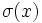# Finitary symmetric group is normal in symmetric group

This article gives the statement, and possibly proof, of a particular subgroup or type of subgroup (namely, Finitary symmetric group (?)) satisfying a particular subgroup property (namely, Normal subgroup (?)) in a particular group or type of group (namely, Symmetric group (?)).

## Statement

Let$S$ be a set. Let$K = \operatorname{Sym}(S)$ be the symmetric group on$S$, and$G$ be the finitary symmetric group on$S$. In other words,$G$ is the subgroup of$K$ comprising the finitary permutations, i.e., the permutations that move only finitely many elements. Then,$G$ is a normal subgroup of$K$.

## Proof

Given: A set$S$.$G = \operatorname{FSym}(S)$ is a subgroup of$K = \operatorname{Sym}(S)$.$\sigma \in K$ and$\alpha \in G$.

To prove:$\sigma\alpha\sigma^{-1} \in G$.

Proof: If$\sigma \in K$ and$\alpha \in G$, we have:$(\sigma\alpha\sigma^{-1})(\sigma(x)) = \sigma(\alpha(x))$.

Thus,$\sigma(x)$ is moved by$\sigma \alpha \sigma^{-1}$ if and$x$ is moved by$\alpha$. Since$\sigma$ is a permutation, this shows that the number of points moved by$\alpha$ and$\sigma\alpha\sigma^{-1}$ is equal. In particular,$\sigma\alpha\sigma^{-1}$ also moves only finitely many points, and hence is in$G$.

Thus,$G$ is normal in$K$.